+91 9404 340 614    gyaanibuddy@gmail.com

0 Likes

#### IEEE: Decision Support System For Determining Inventory And Sales Of Goods Using Economic Order Quantity Methods And Linear Regression

Last updated on April 6, 2021, 8:25 p.m. by tushar

Summary of research paper and important sentences

In the paper titled Decision Support System For Determining Inventory And Sales Of Goods Using Economic Order Quantity Methods And Linear Regression, the authors have made research and development of Decision support systems(DSS) to help make decisions in determining inventory and sales of goods. Also, efficient use of Economic Order Quantity (EOQ) using Linear Regression so as to estimate the amount of quantity each time order of

goods and predict the number of sales in the next period. As, DSS is a computer-based information system it provides interactive information support for managers and

business people during the decision-making process so as to be efficient in purchase of new goods and have minimal loss if in case. So, to meet the features that DSS provides, it uses an analytical model, a specialized database, a decision maker which has insight and judgment,

and a computer-based and interactive model for decisions. Then the EOQ value is calculated. Also, for determining the safety stock factors like time, average raw materials usage, costs used are important ones. Considering these points, a Reorder point is calculated which shows when should a business institution order for materials to maintain stock. After which Linear Regression is applied to test the extent of the causal relationship between the variable causal factors and the effect variable by taking the causative factor is as X (also known as predictor) while the resultant variable as Y (also known as a response). The research method basically includes Development method, Current System Analysis, EOQ method, and lastly Linear Regression. The results are then analysed after which Software Implementation is taken into consideration so as to provide an interface which makes this DSS complete and ready to use.

Important Sentences -

• Decision support system (DSS) is a computer-based information system that provides interactive information support for managers and business people during the decision-making process.
• EOQ is a mathematical method that determines the number of goods that must be ordered to meet the projected demand with minimized supplies.
• Linear regression is a regression analysis that involves the relationship between one dependent variable (sales) and one independent variable (sales period).
• The decision support system uses
• an analytical model
• a specialized database
• a decision maker who has insight and judgment
• a computer-based and interactive model / form making
• process to support business decisions
• The formula for calculating EOQ is as follows: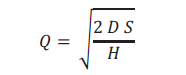• The optimal total cost of the Q value: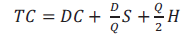• There are basic assumptions in the use of the EOQ model, namely :
• The rate of sales can be estimated
• Constant use of materials
• Orders can be made instantly
• Delivery can be made quickly
• Determining factors in calculating safety stock, are namely:
• Average raw material usage
• The time factor
• Costs used
• Reorder Point is the point at which a company or business institution must order goods or materials, to create controlled inventory conditions. Reorder Point is given by -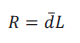• The interval of ordering times in one year is found using :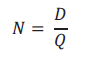• In Linear Regression, The causative factor is generally denoted by X or also known as predictor while the resultant variable is denoted by Y or also known as a response.

Linear Regression Equation: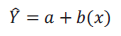To calculate parameters a and b: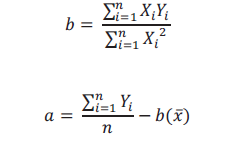• Development method: The development method used is the Prototyping method. Software prototyping is a system life cycle method based on the concept of a working model.
• Current System Analysis: At this time, the running system is still manual. When there is an order, the employee will check the inventory, if the goods run out, it will be recorded as out of stock.
• EOQ: EOQ is a method used to calculate the number of orders That are effective to be efficient in terms of both storage costs and customer costs.
• Linear Regression Method: In this study, the authors estimate the number of sales in the next period.
• The results of the research and development of a decision support system, it can be concluded that this system can calculate the effective amount of inventory with the Economic Order Quantity (EOQ) method, and can estimate the number of sales in the next period using the linear regression method.

0 Likesby tushar
KJ Somaiya College of Engineering Mumbai

Software Engineer | SWE Intern'21 @ConnectWise | Ex- Smollan | KJSCE CSE'22#### Suggested PostsHow I scored 333 in GRE

Feb. 24, 2021, 10:20 a.m.Data- It is getting bigger !

Jan. 27, 2021, 12:26 p.m.Making Extensions in Chrome.

Jan. 27, 2021, 12:06 p.m.